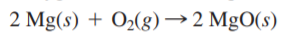×
Get Full Access to Chemistry: A Molecular Approach - 3 Edition - Chapter 4 - Problem 50e
Get Full Access to Chemistry: A Molecular Approach - 3 Edition - Chapter 4 - Problem 50e

×

# Magnesium oxide can be made by heating magnesium metal inISBN: 9780321809247 1

## Solution for problem 50E Chapter 4

Chemistry: A Molecular Approach | 3rd Edition

• Textbook Solutions
• 2901 Step-by-step solutions solved by professors and subject experts
• Get 24/7 help from StudySoup virtual teaching assistantsChemistry: A Molecular Approach | 3rd Edition

4 5 1 364 Reviews
18
3
Problem 50E

Magnesium oxide can be made by heating magnesium metal in the presence of oxygen. The balanced equation for the reaction isWhen 10.1 g of Mg reacts with 10.5 g O2, 11.9 g MgO is collected. Determine the limiting reactant, theoretical yield, and percent yield for the reaction.

Step-by-Step Solution:
Step 1 of 3

Solution: Here, we are going to determine the oxidation number of the indicated element in the given set of compounds. Step1: Oxidation number denotes the oxidation state of an element in a compound ascertained according to a set of rules. These rules are: 1. In elements, in the free or the uncombined state, each atom bears an oxidation number of zero. Evidently each atom in H2, O2, Cl2, O3, P4, S8, Na, Mg, Al has the oxidation number zero. 2. For ions composed of only one atom, the oxidation number is equal to the charge on the ion. In their compounds all alkali metals have oxidation number of +1, and all alkaline earth metals have an oxidation number of +2. Aluminium is regarded to have an oxidation number of +3 in all its compounds. 3. The oxidation number of oxygen in most compounds is –2. 4. The oxidation number of hydrogen is +1, except when it is bonded to metals in binary compounds (that is compounds containing two elements). 5. In all its compounds, fluorine has an oxidation number of –1. Other halogens (Cl, Br,and I) also have an oxidation number of –1, when they occur as halide ions in their compounds. 6. The algebraic sum of the oxidation number of all the atoms in a compound must be zero. In polyatomic ion, the algebraic sum of all the oxidation numbers of atoms of the ion must equal the charge on the ion. Step2: Based upon these rules, let us calculate the oxidation number of the indicated elements in the given compounds: a) Co in LiCoO 2 Since, LiCoO i2 neutral molecule, so, sum of oxidation numbers of all its atoms will be zero. Thus, (+1) + x + 2(-2) = 0 [x being the oxidation number of Co] x +1 - 4 = 0 x - 3 = 0 x = +3 Thus, the oxidation number of Co in LiCoO is +2 b) Al in NaAIH 4 Since, NaAIH is a neutral molecule, so, sum of oxidation numbers of all its atoms will be zero. 4 Thus, (+1) + x + 4(-1) = 0 [x being the oxidation number of Al] x +1 - 4 = 0 x - 3 = 0 x = +3 Thus, the oxidation number of Al in NaAIH is +3. 4 c) C in CH OH3methanol) Since, CH OH is a neutral molecule, so, sum of oxidation numbers of all its atoms will be zero. 3 Thus, x + 3(+1) + (-2) + (+1) = 0 [x being the oxidation number of C] x +3 - 2 + 1 = 0 x + 2 = 0 x = -2 Thus, the oxidation number of C in CH OH is -2. 3 d) N in GaN Since, GaN is neutral molecule, so, sum of oxidation numbers of all its atoms will be zero. Thus, (+3) + x = 0 [x being the oxidation number of N] x = -3 Thus, the oxidation number of N in GaN is -3. e) Cl in HCIO 2 Since, HCIO is 2 eutral molecule, so, sum of oxidation numbers of all its atoms will be zero. Thus, (+1) + x + 2(-2) = 0 [x being the oxidation number of Cl] x +1 - 4 = 0 x - 3 = 0 x = +3 Thus, the oxidation number of Cl in HCIO is +3. 2 f) Cr in BaCr0 4 Since, BaCr0 is a neutral molecule, so, sum of oxidation numbers of all its atoms will be zero. 4 Thus, (+2) + x + 4(-2) = 0 [x being the oxidation number of Cr] x +2 - 8 = 0 x - 6 = 0 x = +6 Thus, the oxidation number of Cr in BaCr0 is +6. 4 --------------

Step 2 of 3

Step 3 of 3

##### ISBN: 9780321809247

Unlock Textbook Solution